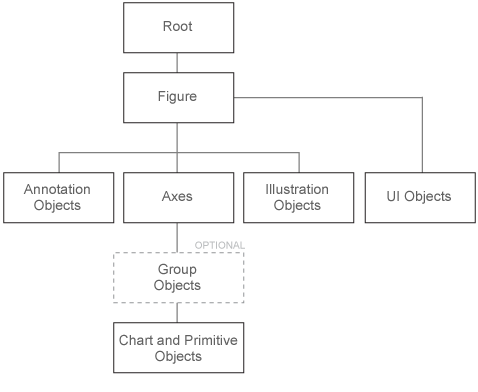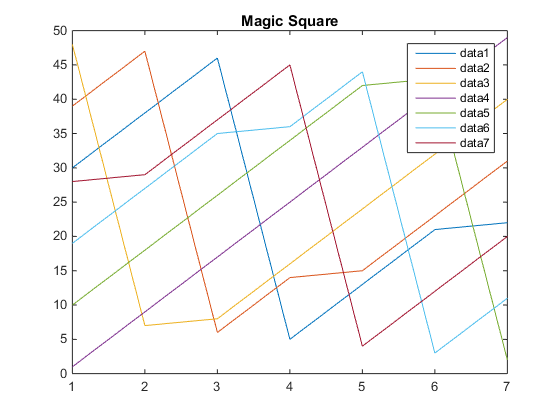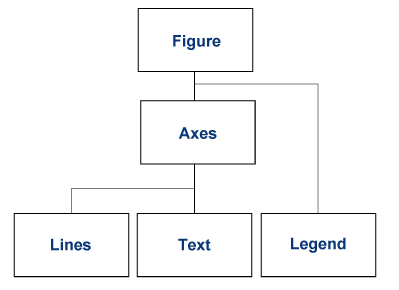## Graphics Object Hierarchy

### MATLAB Graphics Objects

Graphics objects are the visual components used by MATLAB® to display data graphically. For example, a graph can contain lines, text, and axes, all displayed in a figure window.

Each object has a unique identifier called a handle. Using this handle, you can manipulate the characteristics of an existing graphics object by setting object properties. You can also specify values for properties when you create a graphics object. Typically, you create graphics objects using plotting functions like `plot`, `bar`, `scatter`, and so on.

### Graphs Are Composed of Specific Objects

When you create a graph, for example by calling the `plot` function, MATLAB automatically performs a number of steps to produce the graph. These steps involve creating objects and setting the properties of these objects to appropriate values for your specific graph.

### Organization of Graphics Objects

Graphics objects are organized into a hierarchy, as shown by the following diagram.The hierarchical nature of graphics objects reflects the containment of objects by other objects. Each object plays a specific role in the graphics display.

For example, suppose you create a line graph with the `plot` function. An axes object defines a frame of reference for the lines that represent data. A figure is the window to display the graph. The figure contains the axes and the axes contains the lines, text, legends, and other objects used to represent the graph.

Note

An axes is a single object that represents x-, y-, and z-axis scales, tick marks, tick labels, axis labels, and so on.

Here is a simple graph.This graph forms a hierarchy of objects.#### Parent-Child Relationship

The relationship among objects is held in the `Parent` and `Children` properties. For example, the parent of an axes is a figure. The `Parent` property of an axes contains the handle to the figure in which it is contained.

Similarly, the `Children` property of a figure contains any axes that the figure contains. The figure `Children` property also contains the handles of any other objects it contains, such as legends and user-interface objects.

You can use the parent-child relationship to find object handles. For example, if you create a plot, the current axes `Children` property contains the handles to all the lines:

```plot(rand(5)) ax = gca; ax.Children ```
```ans = 5x1 Line array: Line Line Line Line Line```

You can also specify the parent of objects. For example, create a group object and parent the lines from the axes to the group:

```hg = hggroup; plot(rand(5),'Parent',hg)```